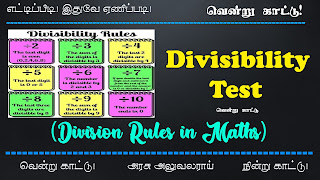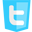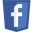Home » , , » Divisibility Test (Division Rules in Maths)

### Divisibility Test (Division Rules in Maths)

Divisibility Test (Division Rules in Maths)Divisibility tests
Divisibility tests in Maths help one to check whether a number is divisible by another number without actual performing division. If a number is exactly divisible by another number it means that in such a case quotient will be a whole number and the division will leave zero as remainder. But not every number is exactly divisible by every other number. In such cases, they leave remainder other than zero. So, thus these rules play a vital role in helping us to determine the actual divisor of a number just by considering the digits of the number. Let us learn these rules for different whole numbers.

Divisibility by 1

Every number is divisible by 1. Divisibility rule for 1 doesn’t have any particular condition. .

Divisibility by 2

All even numbers or any number whose last digit is an even number i.e.  2,4,6,8 including 0 is always exactly divisible by 2.

Divisibility Rules for 3

Divisibility rule for 3 states that a number is exactly divisible by 3 if the sum of its digits is divisible by 3 i.e., it is a multiple of 3

Divisibility by 4

If the last two digits of a number are divisible by 4, then that number is a multiple of 4 and is divisible by 4 exactly.

Divisibility by 5

Numbers with last digit 0 or 5 are always divisible by 5.

Divisibility by 6

Numbers which are divisible by both 2 and 3 are divisible by 6. That is, if last digit of the given number is even and the sum of its digits is a multiple of 3, then the given number is also a multiple of 6 and exactly divisible by 8.

Divisibility Rules for 7

The rule for divisibility by 7 is given below:
Double the last digit and subtract it from the remaining leading truncated number. If the result is divisible by 7, then so was the original number. Apply this rule over and over again as necessary. Example: 826. Twice 6 is 12. So take 12 from the truncated 82. Now 82-12=70. This is divisible by 7, so 826 is divisible by 7 also.

Divisibility by 8

If the last three digits of a number are divisible by 8, then the number is exactly divisible by 8.

Divisibility by 9

The rule for divisibility by 9 is similar to divisibility rule for 3. That is, if the sum of digits of the number is divisible by 9, then the number itself is divisible by 9.

Divisibility by 10

The rule for divisibility by 10 is that for any number whose last digit is 0, it is divisible by 10.

Divisibility Rules for 11

If the difference of the sum of alternative digits of a number is divisible by 11 or zero then that number is divisible by 11 completely.

In order to check whether a number like 11121 is divisible by 11 following is the procedure.

Group the alternative digits i.e. digits which are in odd places together and digits in even places together. Here 111 and 12 are two groups.
Take the sum of the digits of each group i.e. 1+1+1=3 and 1+2= 3
Now find the difference of the sums; 3-3=0
If the difference is zero / divisible by 11, then the original number is also divisible by 11. Here 0 is the difference.
Therefore, 11121 is divisible by 11.

In order to check whether a number like 7145 is divisible by 11 following is the procedure.
Group the alternative digits i.e. digits which are in odd places together and digits in even places together. Here 74 and 15 are two groups.
Take the sum of the digits of each group i.e. 7+4=11 and 1+5= 6
Now find the difference of the sums; 11-6=5

If the difference is divisible by 11, then the original number is also divisible by 11. Here 5 is the difference which is not divisible by 11.
Therefore, 7145 is not divisible by 11.

Divisibility by 12

If a number is divisible by both 3 and 4, then it is divisible by 12.

Divisibility Rules for 13

Test for divisibility by 13. Add four times the last digit to the remaining leading truncated number. If the result is divisible by 13, then so was the first number. Apply this rule over and over again as necessary.
Example: 50661                 =>5066+4=5070
=>507+0=507
=>50+28=78 and 78 is a multiple of 13 (i.e., 78= 6x13), so 50661 is divisible by 13.

Divisibility by 15

If a number is divisible by both 3 and 5, then it is divisible by 15.
Divisibility by 18

If a number is divisible by both 2 and 9, then it is divisible by 18.

Divisibility by 25

In a number, if the last two digits are zeroes or the number formed by the last two digits is a multiple of 25, then the number is divisible by 25.புதிய பதிவுகளை ஈமெயிலில் பெற

## Featured Post

### Tnpsc - ஐந்தாண்டு திட்டங்கள் - இந்தியப் பொருளாதாரம் - Indian Economics

1930 ஆம் ஆண்டுகளில் ஆங்கிலேயர் ஆட்சிக் காலகட்டத்தில் இந்திய அரசியல் அறிவு சார்ந்தவர்கள் இடையே இந்திய பொருளாதார வளர்ச்சிக்கான திட்டமிடல் கு...## Popular Posts

Support : Creating Website | Johny Template | Mas Template